Global Academy of Life Science CSIR-UGC (JRF-NET)

*Free Online Test Series*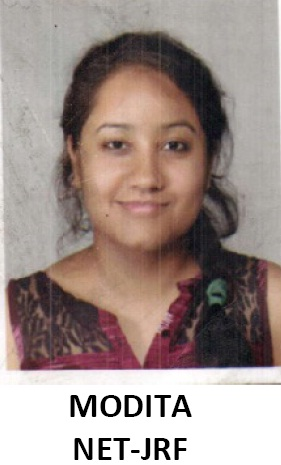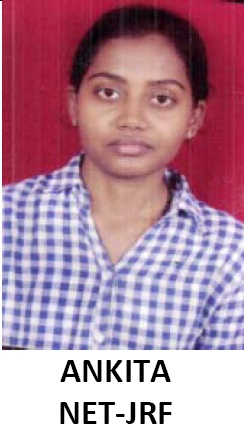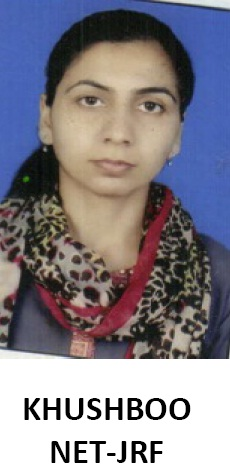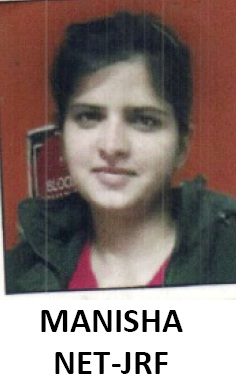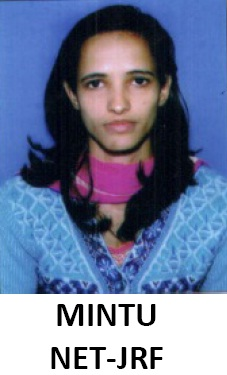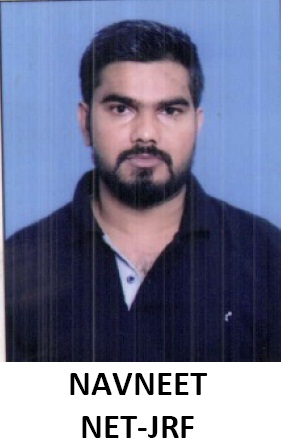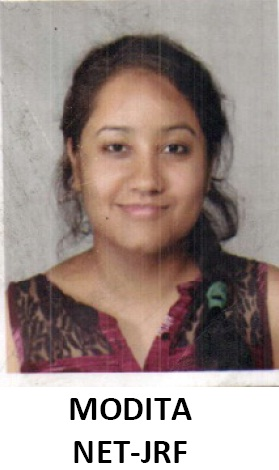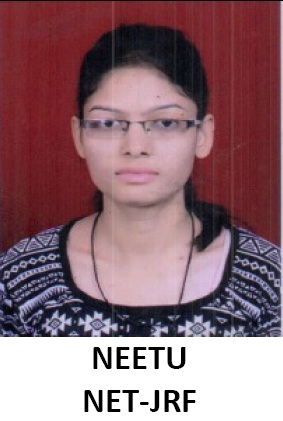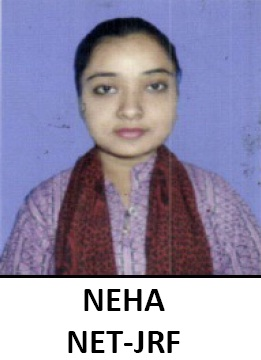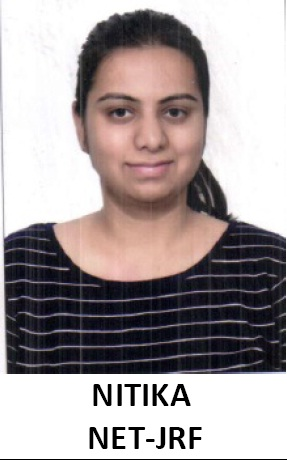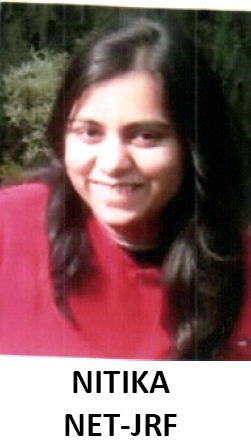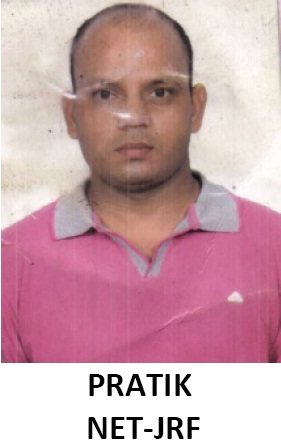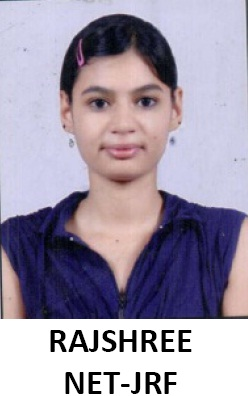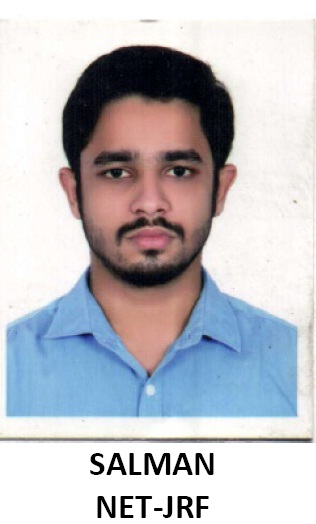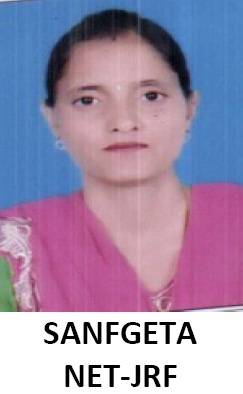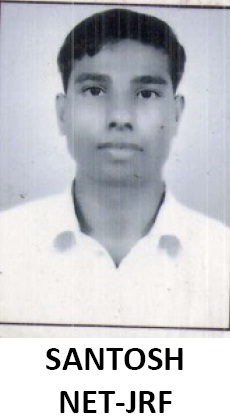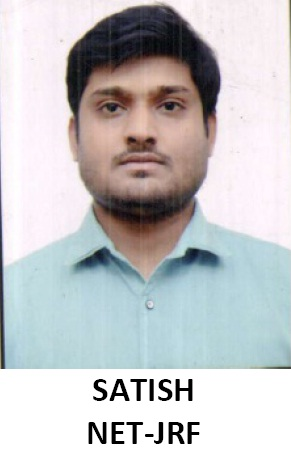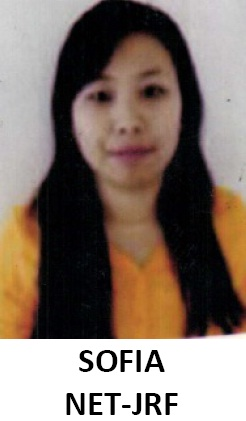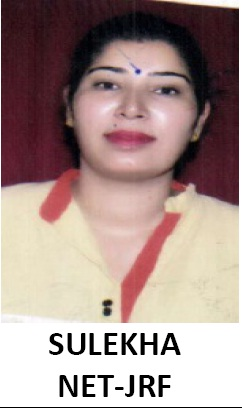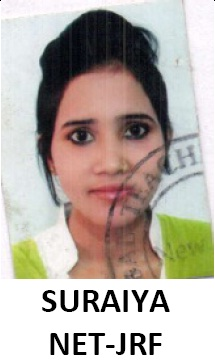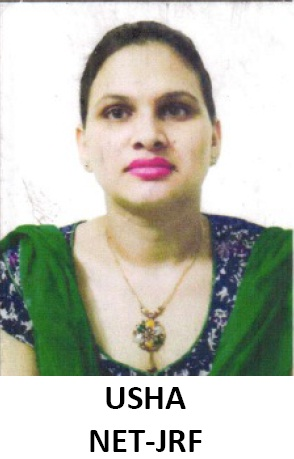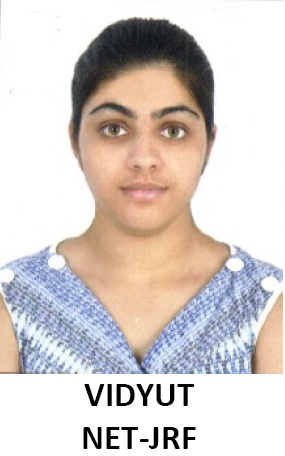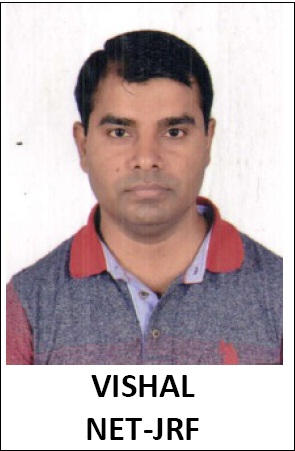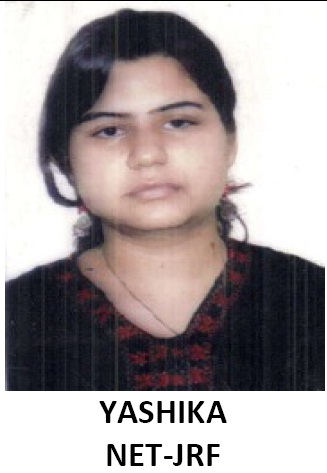•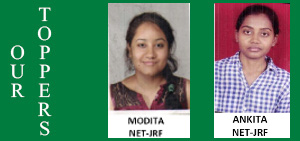•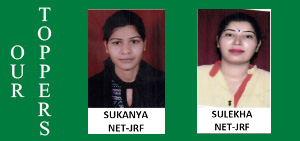•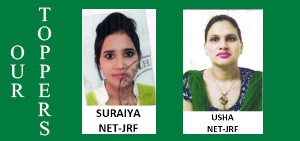•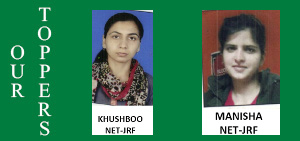•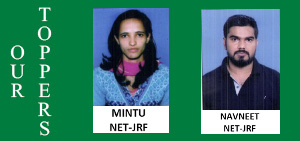•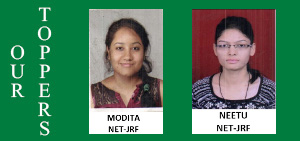•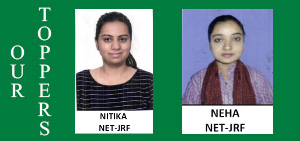•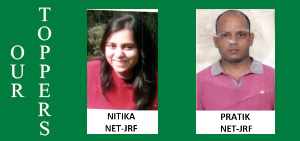•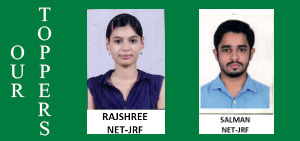•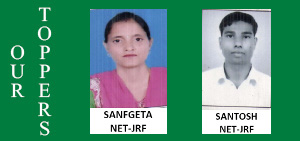•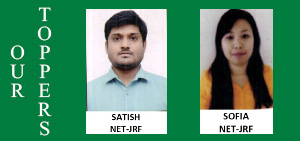Quick Contact

 Name Query Contact Email

PHYSICAL SCIENCE

Council of Scientific and Industrial Research Human Resource Development Group Examination Unit

CSIR-UGC (NET) EXAM FOR AWARD OF JUNIOR RESEARCH FELLOWSHIP AND ELIGIBILITY FOR LECTURERSHIP

PHYSICAL SCIENCES

EXAM SCHEME

TIME: 3 HOURS

MAXIMUM MARKS: 200

CSIR-UGC (NET) Exam for Award of Junior Research Fellowship and Eligibility for Lecturership shall be a Single Paper Test having Multiple Choice Questions (MCQs). The question paper is divided in three parts

Part 'A' This part shall carry 20 questions pertaining to General Science, Quantitative Reasoning & Analysis and Research Aptitude. The candidates shall be required to answer any 15 questions. Each question shall be of two marks. The total marks allocated to this section shall be 30 out of 200.

Part 'B' This part shall contain 25 Multiple Choice Questions (MCQs) generally covering the topics given in the Part ‘A’ (CORE) of syllabus. Each question shall be of 3.5 Marks. The total marks allocated to this section shall be 70 out of 200.Candidates are required to answer any 20 questions.

Part 'C' This part shall contain 30 questions from Part ‘B’ (Advanced) and Part ‘A’ that are designed to test a candidate's knowledge of scientific concepts and/or application of the scientific concepts. The questions shall be of analytical nature where a candidate is expected to apply the scientific knowledge to arrive at the solution to the given scientific problem. A candidate shall be required to answer any 20. Each question shall be of 5 Marks. The total marks allocated to this section shall be 100 out of 200.

There will be negative marking @25% for each wrong answer.

Model Question Paper is available on HRDG website www.csirhrdg.res.in

CSIR-UGC National Eligibility Test (NET) for Junior Research Fellowship and Lecturer-ship

PHYSICAL SCIENCE

PART ‘A’ CORE

A. Mathematical Methods of Physics : Dimensional analysis. Vector algebra and vector calculus. Linear algebra, matrices, Cayley-Hamilton Theorem. Eigenvalues and eigenvectors. Linear ordinary differential equations of first & second order, Special functions (Hermite, Bessel, Laguerre and Legendre functions). Fourier series, Fourier and Laplace transforms. Elements of complex analysis, analytic functions; Taylor & Laurent series; poles, residues and evaluation of integrals. Elementary probability theory, random variables, binomial, Poisson and normal distributions. Central limit theorem

B. Classical Mechanics :Newton’s laws. Dynamical systems, Phase space dynamics, stability analysis. Central force motions. Two body Collisions - scattering in laboratory and Centre of mass frames. Rigid body dynamicsmoment of inertia tensor. Non-inertial frames and pseudoforces. Variational principle. Generalized coordinates. Lagrangian and Hamiltonian formalism and equations of motion. Conservation laws and cyclic coordinates. Periodic motion: small oscillations, normal modes. Special theory of relativityLorentz transformations, relativistic kinematics and mass–energy equivalence.

C. Electromagnetic Theory : Electrostatics: Gauss’s law and its applications, Laplace and Poisson equations, boundary value problems. Magnetostatics: Biot-Savart law, Ampere's theorem. Electromagnetic induction. Maxwell's equations in free space and linear isotropic media; boundary conditions on the fields at interfaces. Scalar and vector potentials, gauge invariance. Electromagnetic waves in free space. Dielectrics and conductors. Reflection and refraction, polarization, Fresnel’s law, interference, coherence, and diffraction. Dynamics of charged particles in static and uniform electromagnetic fields.

D. Quantum Mechanics : Wave-particle duality. Schrödinger equation (time-dependent and time-independent). Eigenvalue problems (particle in a box, harmonic oscillator, etc.). Tunneling through a barrier. Wave-function in coordinate and momentum representations. Commutators and Heisenberg uncertainty principle. Dirac notation for state vectors. Motion in a central potential: orbital angular momentum, angular momentum algebra, spin, addition of angular momenta; Hydrogen atom. Stern-Gerlach experiment. Timeindependent perturbation theory and applications. Variational method. Time dependent perturbation theory and Fermi's golden rule, selection rules. Identical particles, Pauli exclusion principle, spin-statistics connection.

E. Thermodynamic and Statistical Physics : Laws of thermodynamics and their consequences. Thermodynamic potentials, Maxwell relations, chemical potential, phase equilibria. Phase space, micro- and macro-states. Micro-canonical, canonical and grand-canonical ensembles and partition functions. Free energy and its connection with thermodynamic quantities. Classical and quantum statistics. Ideal Bose and Fermi gases. Principle of detailed balance. Blackbody radiation and Planck's distribution law.

F. Electronics and Experimental Methods : Semiconductor devices (diodes, junctions, transistors, field effect devices, homo- and hetero-junction devices), device structure, device characteristics, frequency dependence and applications. Opto-electronic devices (solar cells, photo-detectors, LEDs). Operational amplifiers and their applications. Digital techniques and applications (registers, counters, comparators and similar circuits). A/D and D/A converters. Microprocessor and microcontroller basics.
Data interpretation and analysis. Precision and accuracy. Error analysis, propagation of errors. Least squares fitting,

A. Mathematical Methods of Physics : Green’s function. Partial differential equations (Laplace, wave and heat equations in two and three dimensions). Elements of computational techniques: root of functions, interpolation, extrapolation, integration by trapezoid and Simpson’s rule, Solution of first order differential equation using RungeKutta method. Finite difference methods. Tensors. Introductory group theory: SU(2), O(3).

B. Classical Mechanics: Dynamical systems, Phase space dynamics, stability analysis. Poisson brackets and canonical transformations. Symmetry, invariance and Noether’s theorem. Hamilton-Jacobi theory.

C. Electromagnetic Theory: Dispersion relations in plasma. Lorentz invariance of Maxwell’s equation. Transmission lines and wave guides. Radiation- from moving charges and dipoles and retarded potentials.

D. Quantum Mechanics : Spin-orbit coupling, fine structure. WKB approximation. Elementary theory of scattering: phase shifts, partial waves, Born approximation. Relativistic quantum mechanics: Klein-Gordon and Dirac equations. Semi-classical theory of radiation.

E. Thermodynamic and Statistical Physics: First- and second-order phase transitions. Diamagnetism, paramagnetism, and ferromagnetism. Ising model. Bose-Einstein condensation. Diffusion equation. Random walk and Brownian motion. Introduction to nonequilibrium processes.

F. Electronics and Experimental Methods: Linear and nonlinear curve fitting, chi-square test. Transducers (temperature, pressure/vacuum, magnetic fields, vibration, optical, and particle detectors). Measurement and control. Signal conditioning and recovery. Impedance matching, amplification (Op-amp based, instrumentation amp, feedback), filtering and noise reduction, shielding and grounding. Fourier transforms, lock-in detector, box-car integrator, modulation techniques.
High frequency devices (including generators and detectors).

G. Atomic & Molecular Physics : Quantum states of an electron in an atom. Electron spin. Spectrum of helium and alkali atom. Relativistic corrections for energy levels of hydrogen atom, hyperfine structure and isotopic shift, width of spectrum lines, LS & JJ couplings. Zeeman, Paschen-Bach & Stark effects. Electron spin resonance. Nuclear magnetic resonance, chemical shift. Frank-Condon principle. Born-Oppenheimer approximation. Electronic, rotational, vibrational and Raman spectra of diatomic molecules, selection rules. Lasers: spontaneous and stimulated emission, Einstein A & B coefficients. Optical pumping, population inversion, rate equation. Modes of resonators and coherence length.

H. Condensed Matter Physics: Bravais lattices. Reciprocal lattice. Diffraction and the structure factor. Bonding of solids. Elastic properties, phonons, lattice specific heat. Free electron theory and electronic specific heat. Response and relaxation phenomena. Drude model of electrical and thermal conductivity. Hall effect and thermoelectric power. Electron motion in a periodic potential, band theory of solids: metals, insulators and semiconductors. Superconductivity: type-I and type-II superconductors. Josephson junctions. Superfluidity. Defects and dislocations. Ordered phases of matter: translational and orientational order, kinds of liquid crystalline order. Quasi crystals

I. Nuclear and Particle Physics: Basic nuclear properties: size, shape and charge distribution, spin and parity. Binding energy, semiempirical mass formula, liquid drop model. Nature of the nuclear force, form of nucleon-nucleon potential, charge-independence and charge-symmetry of nuclear forces. Deuteron problem. Evidence of shell structure, single-particle shell model, its validity and limitations. Rotational spectra. Elementary ideas of alpha, beta and gamma decays and their selection rules. Fission and fusion. Nuclear reactions, reaction mechanism, compound nuclei and direct reactions.

Classification of fundamental forces. Elementary particles and their quantum numbers (charge, spin, parity, isospin, strangeness, etc.). Gellmann-Nishijima formula. Quark model, baryons and mesons. C, P, and T invariance. Application of symmetry arguments to particle reactions. Parity non-conservation in weak interaction. Relativistic kinematics.